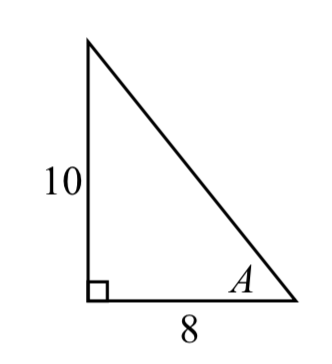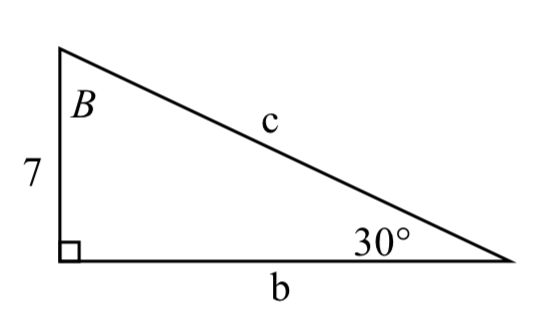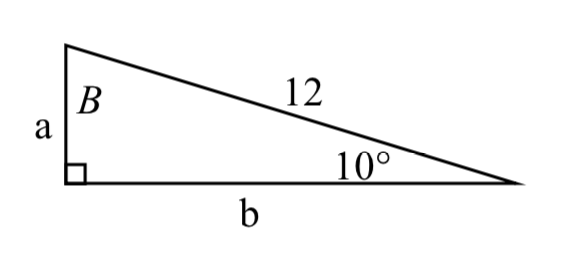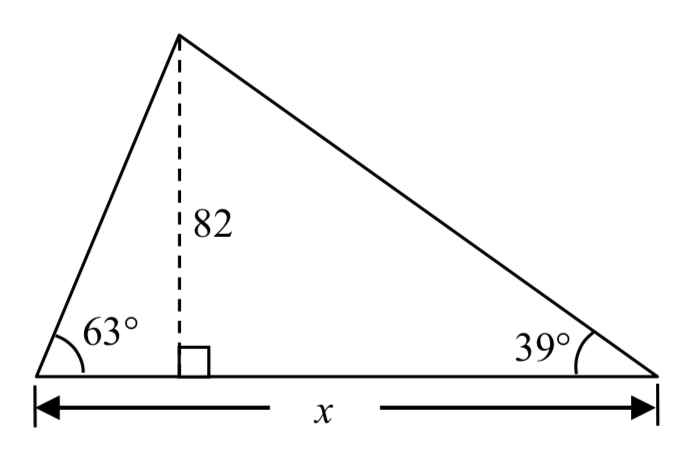$$\newcommand{\id}{\mathrm{id}}$$ $$\newcommand{\Span}{\mathrm{span}}$$ $$\newcommand{\kernel}{\mathrm{null}\,}$$ $$\newcommand{\range}{\mathrm{range}\,}$$ $$\newcommand{\RealPart}{\mathrm{Re}}$$ $$\newcommand{\ImaginaryPart}{\mathrm{Im}}$$ $$\newcommand{\Argument}{\mathrm{Arg}}$$ $$\newcommand{\norm}{\| #1 \|}$$ $$\newcommand{\inner}{\langle #1, #2 \rangle}$$ $$\newcommand{\Span}{\mathrm{span}}$$

# 5.5.5E: Right Triangle Trigonometry (Exercises)

$$\newcommand{\vecs}{\overset { \rightharpoonup} {\mathbf{#1}} }$$ $$\newcommand{\vecd}{\overset{-\!-\!\rightharpoonup}{\vphantom{a}\smash {#1}}}$$$$\newcommand{\id}{\mathrm{id}}$$ $$\newcommand{\Span}{\mathrm{span}}$$ $$\newcommand{\kernel}{\mathrm{null}\,}$$ $$\newcommand{\range}{\mathrm{range}\,}$$ $$\newcommand{\RealPart}{\mathrm{Re}}$$ $$\newcommand{\ImaginaryPart}{\mathrm{Im}}$$ $$\newcommand{\Argument}{\mathrm{Arg}}$$ $$\newcommand{\norm}{\| #1 \|}$$ $$\newcommand{\inner}{\langle #1, #2 \rangle}$$ $$\newcommand{\Span}{\mathrm{span}}$$ $$\newcommand{\id}{\mathrm{id}}$$ $$\newcommand{\Span}{\mathrm{span}}$$ $$\newcommand{\kernel}{\mathrm{null}\,}$$ $$\newcommand{\range}{\mathrm{range}\,}$$ $$\newcommand{\RealPart}{\mathrm{Re}}$$ $$\newcommand{\ImaginaryPart}{\mathrm{Im}}$$ $$\newcommand{\Argument}{\mathrm{Arg}}$$ $$\newcommand{\norm}{\| #1 \|}$$ $$\newcommand{\inner}{\langle #1, #2 \rangle}$$ $$\newcommand{\Span}{\mathrm{span}}$$

Section 5.5 Exercise

Note: pictures may not be drawn to scale.

In each of the triangles below, find $$\sin \left(A\right),\cos \left(A\right),\tan \left(A\right),\sec \left(A\right),\csc \left(A\right),\cot \left(A\right)$$.

1.2.In each of the following triangles, solve for the unknown sides and angles.

3.4.5.6.7.8.9. A 33-ft ladder leans against a building so that the angle between the ground and the ladder is 80$$\mathrm{{}^\circ}$$. How high does the ladder reach up the side of the building?

10. A 23-ft ladder leans against a building so that the angle between the ground and the ladder is 80$$\mathrm{{}^\circ}$$. How high does the ladder reach up the side of the building?

11. The angle of elevation to the top of a building in New York is found to be 9 degrees from the ground at a distance of 1 mile from the base of the building. Using this information, find the height of the building.

12. The angle of elevation to the top of a building in Seattle is found to be 2 degrees from the ground at a distance of 2 miles from the base of the building. Using this information, find the height of the building.

13. A radio tower is located 400 feet from a building. From a window in the building, a person determines that the angle of elevation to the top of the tower is 36$$\mathrm{{}^\circ}$$ and that the angle of depression to the bottom of the tower is 23$$\mathrm{{}^\circ}$$. How tall is the tower?

14. A radio tower is located 325 feet from a building. From a window in the building, a person determines that the angle of elevation to the top of the tower is 43$$\mathrm{{}^\circ}$$ and that the angle of depression to the bottom of the tower is 31$$\mathrm{{}^\circ}$$. How tall is the tower?

15. A 200 foot tall monument is located in the distance. From a window in a building, a person determines that the angle of elevation to the top of the monument is 15$$\mathrm{{}^\circ}$$ and that the angle of depression to the bottom of the tower is 2$$\mathrm{{}^\circ}$$. How far is the person from the monument?

16. A 400 foot tall monument is located in the distance. From a window in a building, a person determines that the angle of elevation to the top of the monument is 18$$\mathrm{{}^\circ}$$ and that the angle of depression to the bottom of the tower is 3$$\mathrm{{}^\circ}$$. How far is the person from the monument?

17. There is an antenna on the top of a building. From a location 300 feet from the base of the building, the angle of elevation to the top of the building is measured to be 40$$\mathrm{{}^\circ}$$. From the same location, the angle of elevation to the top of the antenna is measured to be 43$$\mathrm{{}^\circ}$$. Find the height of the antenna.

18. There is lightning rod on the top of a building. From a location 500 feet from the base of the building, the angle of elevation to the top of the building is measured to be 36$$\mathrm{{}^\circ}$$. From the same location, the angle of elevation to the top of the lightning rod is measured to be 38$$\mathrm{{}^\circ}$$. Find the height of the lightning rod.

19. Find the length $$x$$.20. Find the length $$x$$.21. Find the length $$x$$.22. Find the length $$x$$.23. A plane is flying 2000 feet abovesea level toward a mountain. The pilot observes the top of the mountain to be 18$${}^{\circ}$$ above the horizontal, then immediately flies the plane at an angle of 20$${}^{\circ}$$ above horizontal. The airspeed of the plane is 100 mph. After 5 minutes, the plane is directly above the top of the mountain. How high is the plane above the top of the mountain (when it passes over)? What is the height of the mountain? [UW]

24. Three airplanes depart SeaTac Airport. A United flight is heading in a direction 50$$\mathrm{{}^\circ}$$ counterclockwise from east, an Alaska flight is heading 115$$\mathrm{{}^\circ}$$ counterclockwise from east and a Delta flight is heading 20$$\mathrm{{}^\circ}$$ clockwise from east. [UW]

a. Find the location of the United flight when it is 20 miles north of SeaTac.
b. Find the location of the Alaska flight when it is 50 miles west of SeaTac.
c. Find the location of the Delta flight when it is 30 miles east of SeaTac.25. The crew of a helicopter needs to land temporarily in a forest and spot a flat piece of ground (a clearing in the forest) as a potential landing site, but are uncertain whether it is wide enough. They make two measurements from A (see picture) finding $$\alpha$$ = 25$$\mathrm{{}^\circ}$$ and $$\beta$$ = 54$$\mathrm{{}^\circ}$$. They rise vertically 100 feet to B and measure $$\gamma$$ = 47$$\mathrm{{}^\circ}$$. Determine the width of the clearing to the nearest foot. [UW]26. A Forest Service helicopter needs to determine the width of a deep canyon. While hovering, they measure the angle $$\gamma$$ = 48$$\mathrm{{}^\circ}$$ at position B (see picture), then descend 400 feet to position A and make two measurements: $$\alpha$$ = 13$$\mathrm{{}^\circ}$$ (the measure of $$\angle$$EAD), $$\beta$$ = 53$$\mathrm{{}^\circ}$$ (the measure of $$\angle$$CAD). Determine the width of the canyon to the nearest foot. [UW]1. $$\sin(A) = \dfrac{5\sqrt{41}}{41}$$, $$\cos(A) = \dfrac{4\sqrt{41}}{41}$$, $$\tan(A) = \dfrac{5}{4}$$
$$\sec(A) = \dfrac{\sqrt{41}}{4}$$, $$\csc(A) = \dfrac{\sqrt{41}}{5}$$, $$\cot(A) = \dfrac{4}{5}$$

3. $$c = 14$$, $$b = 7\sqrt{3}$$, $$B = 60^{\circ}$$

5. $$a = 5.3171$$, $$c = 11.3257$$, $$A = 28^{\circ}$$

7. $$a = 9.0631$$, $$b = 4.2262$$, $$B = 25^{\circ}$$

9. 32.4987 ft

11. 836.2698 ft

13. 460.4069 ft

15. 660.35 feet

17. 28.025 ft

19. 143.0427

21. 86.6685